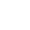## Cost Volume Profit analysis – Formulas

Cost volume profit analysis Overview

• CVP analysis is used to show how costs and profit changes with changes in volume of activity.
• CVP analysis is an application of marginal costing concepts.

Assumptions in CVP analysis

• Costs are either fixed or variable.
• Fixed costs are normally assumed to remain unchanged at all levels of output.
• The contribution per unit is constant for each unit sold. Therefore the contribution to sales ration is also a constant value at all levels of sales.

Contribution

• Contribution is a key concept.
• It is measured as sales revenue (Less) variable costs

Contribution per unit = selling price – variable costs

Contribution/sales ratio (C/S) = contribution per unit
selling price per unit

## Cost Volume Profit (CVP) analysis

• Break-even point is the volume of sales where the profit is ‘zero‘. And the total contribution is exactly equal to the total fixed costs.
• Management wants to know what the break-even point is in order to:
• Identify the minimum volume of sales in order to avoid a loss.
• Asses the amount of risk in budget by comparing the budget volume of sales with break-even volume.
• CVP analysis can be used to calculate a break-even point for sales.In this method Break-even is calculated by using the contribution per unit i.e.;

Break Even = Total fixed costs
Contribution per unit

Once Break-even is calculated as a number of units it is easy to express it in terms of revenue by multiplying no. of units by selling price.

In this method Break-even is calculated by using the contribution to sales ratio (C/S) i.e.;

Break Even = Total fixed costs
C/S ratio

Once Break-even is calculated as in Revenue, it is easy to express it in terms of no. of units by dividing the revenue by selling price per item.• The margin of safety is the difference between:
• Budgeted sales (in units/revenue); and
• Break-even amount of sales (in units/revenue).
• It is usually expressed as a Percentage of the budgeted sales. However it may be measured as;
• A quantity of units (difference between budgeted sales volume and break-even sales volume)
• An amount of sales Revenue (The difference between budgeted sales revenue and total sales revenue required to break-even).
• At margin of safety all fixed cost are ‘0’, any addition would be directly profit.
• It can be positive or negative.
• A high margin of safety indicates a low risk of making a loss.

## Cost Volume Profit (CVP) analysis

• The amount sales must be known in order to achieve a target profit.
• CVP analysis can be used to calculate the volume of sales required.
• The volume of sales required must be sufficient to earn a contribution that covers the fixed costs and make the target amount of profit i,e the contribution needed to earn the target profit is target profit PLUS the fixed costs.

Volume target (units) = Total fixed costs + target profit
Contribution per unit

Volume target (Revenue) = Total fixed costs + target profit
Contribution to sales ratio

## Cost Volume Profit (CVP) analysisFor ‘multi-product cvp analysis’ there is an assumption that products are sold in set ratio which does not change with volume. This assumption allows to calculate weighted average contribution per unit or per batch and contribution to sales (C/S) ratio.Break even in Batches

= Total fixed costs
Contribution per Batch

• Average contribution per unit should be used (multiply sales ratio with contribution per unit of each product and add them)
• Units can be converted into money value by multiplying with sales price.

Break even in Revenue

= Total fixed costs
C/S ratio per Batch

For C/S ratio;

• calculate contribution per Batch.
• calculate average revenue by multiplying sales ratio with selling price of each product.
• now divide contribution per batch by average revenue to have C/S ratio.
• The margin of safety is calculated in same way as for single product by comparing the budgeted activity level to Break-even activity.
• The Break-even can be compared to the Budgeted activity level using Batches, units, revenues.
• Same as for a single product.
• The necessary contribution to earn the target profit is the target profit PLUS the fixed costs.
• This can be calculated using contribution per unit, batch or C/S ratio.